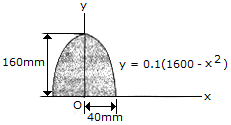# Engineering Mechanics - Moments of Inertia - Discussion

### Discussion :: Moments of Inertia - General Questions (Q.No.1)

1.Determine the radius of gyration ky of the parabolic area.

 [A]. ky = 76.5 mm [B]. ky = 17.89 mm [C]. ky = 78.6 mm [D]. ky = 28.3 mm

Explanation:

No answer description available for this question.

 Prabhu said: (Jul 5, 2011) I want explanation.

 Phani said: (Aug 13, 2011) Can any one explain this?

 Chandu said: (Aug 17, 2011) Hai frnds i solve it........ Ky=root over Iy/A Iy=moment of inertia with respect to y-axis A=area dI=dA*r^2 (r is radius) dI=0.1(1600-x^2)x^2 dx integrate both sides,limits are 0 to 40 and multiple with 2 we get I=2730666 here dA=y dx(here y=0.1(1600-x^2)) integrate both sides, limits are 0 to 40 and multiple with 2 A=8533 substitute the values of I,A in Ky we get Ky=17.89mm

 Crazy Man said: (Aug 3, 2012) Ky=root over Iy/A Iy=moment of inertia with respect to y-axis A=area dI=dA*r^2 (r is radius) dI=0.1(1600-x^2)x^2 dx Integrate both sides, limits are 0 to 40 And multiple with 2 we get I=2730666 Here dA=y dx(here y=0.1(1600-x^2)) Integrate both sides, limits are 0 to 40 And multiple with 2 A=8533 Substitute the values of I, A in Ky we get Ky=17.89mm

 Malik said: (Jun 8, 2014) How that I came?

 Kwesiga Henry said: (May 25, 2015) How is the area of the figure got?

 Sai Deep said: (Oct 26, 2015) Please give me explanation?

 Pradeep said: (Mar 1, 2016) Area of parabola 4ah/3.

 Kumar Saim said: (May 5, 2016) You are correct @Chandu. I'm also tried in the same way, it gives the same answer as yours.

 Akbar Shah said: (Nov 9, 2016) Can anyone provide the simple explanation?

 Abdu said: (Jan 27, 2017) The area of semi-parabola that written in the form of x=k(y^2) or vertical semi-parabola area formula is given by 2*ab/3 so the total area will be 2*(2*ab/3) so 160*80/3= 8533 unit^2 we can take a piece of area dxdy then multiply by x^2 then integrate finally apply gyration formula.